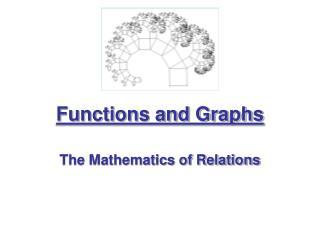DownloadDownload PresentationFunctions and Graphs

# Functions and Graphs

Télécharger la présentation## Functions and Graphs

- - - - - - - - - - - - - - - - - - - - - - - - - - - E N D - - - - - - - - - - - - - - - - - - - - - - - - - - -
##### Presentation Transcript

1. Functions and Graphs The Mathematics of Relations

2. Definition of a Relation

3. Relation (A) (B) • 32 mpg • 8 mpg • 16 mpg (C)

4. Domain and Range • The values that make up the set of independent values are the domain • The values that make up the set of dependent values are the range. • State the domain and range from the 4 examples of relations given.

5. Quick Side Trip Into the Set of Real Numbers

6. The Set of Real Numbers

7. Ponder • To what set does the sum of a rational and irrational number belong? • How many irrational numbers can you generate for each rational number using this fact?

8. Properties of Real Numbers • Transitive:If a = b and b = c then a = c • Identity: a + 0 = a, a • 1 = a • Commutative:a + b = b + a, a • b = b • a • Associative:(a + b) + c = a + (b + c)(a • b) • c = a • (b • c) • Distributive: a(b + c) = ab + aca(b - c) = ab - ac

9. if a is positive if a is negative Definition of Absolute Value

10. The Real Number Line

11. End of Side Trip Into the Set of Real Numbers

12. Definition of a Relation • A Relation maps a value from the domain to the range. A Relation is a set of ordered pairs. • The most common types of relations in algebra map subsets of real numbers to other subsets of real numbers.

13. Example

14. Define the Set of Values that Make Up the Domain and Range. • The relation is the year and the cost of a first class stamp. • The relation is the weight of an animal and the beats per minute of it’s heart. • The relation is the time of the day and the intensity of the sun light. • The relation is a number and it’s square.

15. Definition of a Function • If a relation has the additional characteristic that each element of the domain is mapped to one and only one element of the range then we call the relation a Function.

16. Definition of a Function • If we think of the domain as the set of boys and the range the set of girls, then a function is a monogamous relationship from the domain to the range. Each boy gets to go out with one and only one girl. • But… It does not say anything about the girls. They get to live in Utah.

17. x y RANGE DOMAIN FUNCTION CONCEPT f

18. y1 y2 RANGE NOT A FUNCTION R x DOMAIN

19. x1 y x2 RANGE DOMAIN FUNCTION CONCEPT f

20. X Y 1 1 -5 1 -1 1 3 1 X Y 1 2 -5 7 -1 2 3 3 X Y 1 2 1 7 1 2 1 3 X Y 1 π π 1 -1 5 π 3 Examples • Decide if the following relations are functions.

21. Ponder • Is 0 an even number? • Is the empty set a function?

22. X Y 1 2 5 10 -1 -2 3 6 Ways to Represent a Function • Graphical • Symbolic • Numeric • VerbalThe cost is twice the original amount.

23. Example • Penney’s is having a sale on coats. The coat is marked down 37% from it’s original price at the cash register.

24. If you chose a coat that originally costs \$85.99, what will the sale price be? What amount will you pay in total for the coat (Assume you bought it in California.) • Is this a function? What is the domain and range? Give the symbolic form of the function. If you chose a coat that costs \$C, what will be the amount \$A that you pay for it?

25. Function NotationThe Symbolic Form • A truly excellent notation. It is concise and useful.

26. Name of the function • Output Value• Member of the Range• Dependent Variable These are all equivalent names for the y. • Input Value• Member of the Domain• Independent Variable These are all equivalent names for the x.

27. Example of Function Notation • The f notation

28. Graphical Representation • Graphical representation of functions have the advantage of conveying lots of information in a compact form. There are many types and styles of graphs but in algebra we concentrate on graphs in the rectangular (Cartesian) coordinate system.

29. Average National Price of Gasoline

30. Range Domain Graphs and Functions

31. CBR

32. Vertical Line Test for Functions • If a vertical line intersects a graph once and only once for each element of the domain, then the graph is a function.

33. Big Deal! • A point is in the set of ordered pairs that make up the function if and only if the point is on the graph of the function.

34. Numeric • Tables of points are the most common way of representing a function numerically

35. Verbal • Describing the relation in words. We did this with the opening examples.

36. Key Points • Definition of a function • Ways to represent a functionSymbolicallyGraphicallyNumericallyVerbally